# 11. Numerical integration

Note – The example worksheets are available for download. See 1. How to obtain and use example worksheets.

Examples of numerical integration (quadrature) by XLPack are shown below.

### Example

Let’s compute the following integral.

```
∫ 1/(1 + x2) dx [0, 4]

```

This function is plotted as shown below. The exact value of integral is arctan(4).There are two types of quadrature routines. Function input type computes an integral using user defined function which can compute a function value at any points within integral range. Data input type computes an integral using given data at some points including integral range.

### Solving problem with VBA program (function input) (1)

The following is an example code using VBA library subroutine Qag and Qk15.

Qag is an adaptive quadrature which uses quadrature rule on adaptively refined subintervals of the integration interval and computes the integral value satisfying given target accuracy. Qk15 is the quadrature with fixed abscissae (15 points) and computes the integral and its estimated error using 15 function evaluations.

``````Function F(X As Double) As Double
F = 1 / (1 + X ^ 2)
End Function

Sub Start()
Dim A As Double, B As Double, S As Double, Info As Long
Dim AbsErr As Double, Neval As Long
'--- Input data
A = Cells(4, 2): B = Cells(5, 2)
'--- Compute integration by Qag
Call Qag(AddressOf F, A, B, S, Info, AbsErr, Neval)
Cells(11, 2) = Info
If Info = 0 Then
Cells(8, 2) = S
Cells(9, 2) = AbsErr
Cells(10, 2) = Neval
End If
'--- Compute integration by Qk15
Call Qk15(AddressOf F, A, B, S, AbsErr)
Cells(8, 3) = S
Cells(9, 3) = AbsErr
End Sub``````

You should define the object function f(x) as the external subroutine and call Qag and Qk15 with specifying the integration interval and other parameters if necessary.

The following result is obtained by running this program.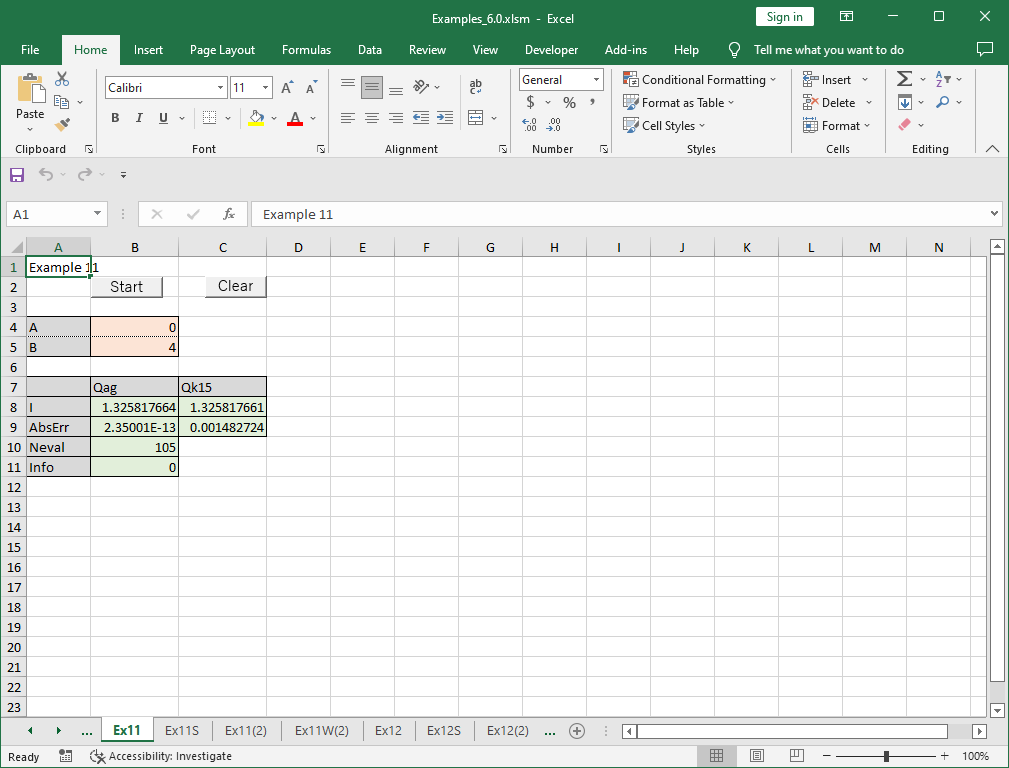Qag computed correct result by 105 function evaluations with maximum accuracy (correct 15 digits). Qk15 returned an estimated error 0.00148 by 15 function evaluations but actually 9 digits are correct.

The adaptive quadrature is appropriate when some specific accuracy is required. The quadrature with fixed abscissae is appropriate for fast computation with certain degree of accuracy.

### Solving problem with VBA program (function input) (2)

The following is an example code using the reverse communication version VBA library subroutine Qag_r and Qk15_r.

``````Sub Start()
Dim A As Double, B As Double, S As Double, Info As Long
Dim AbsErr As Double, Neval As Long
Dim XX As Double, YY As Double, IRev As Long
'--- Input data
A = Cells(4, 2): B = Cells(5, 2)
'--- Compute integration by Qag_r
IRev = 0
Do
Call Qag_r(A, B, S, Info, XX, YY, IRev, AbsErr, Neval)
If IRev = 1 Then YY = 1 / (1 + XX ^ 2)
Loop While IRev <> 0
Cells(11, 2) = Info
If Info = 0 Then
Cells(8, 2) = S
Cells(9, 2) = AbsErr
Cells(10, 2) = Neval
End If
'--- Compute integration by Qk15_r
IRev = 0
Do
Call Qk15_r(A, B, S, XX, YY, IRev, AbsErr)
If IRev = 1 Then YY = 1 / (1 + XX ^ 2)
Loop While IRev <> 0
Cells(8, 3) = S
Cells(9, 3) = AbsErr
End Sub``````

Instead of defining the object function as the external function, when returned with IRev = 1, you need to compute the object function value at XX, enter it in YY and call Qag_r or Qk15_r again. For further details of reverse communication version, see here.

The same result with above is obtained by this program.

### Solving problem with worksheet function (data input)

As the data input quadrature, the method using spline interpolation is described below.

Suppose that the function form is unknown but the function values at x = 0, 0.5, 1, …, 4 are given for the same example above.

x f(x)
0 1
0.5 0.8
1 0.5
1.5 0.3076923
2 0.2
2.5 0.137931
3 0.1
3.5 0.0754717
4 0.0588235
Firstly compute spline coefficients using worksheet function WPchse (see “5. Interpolation”).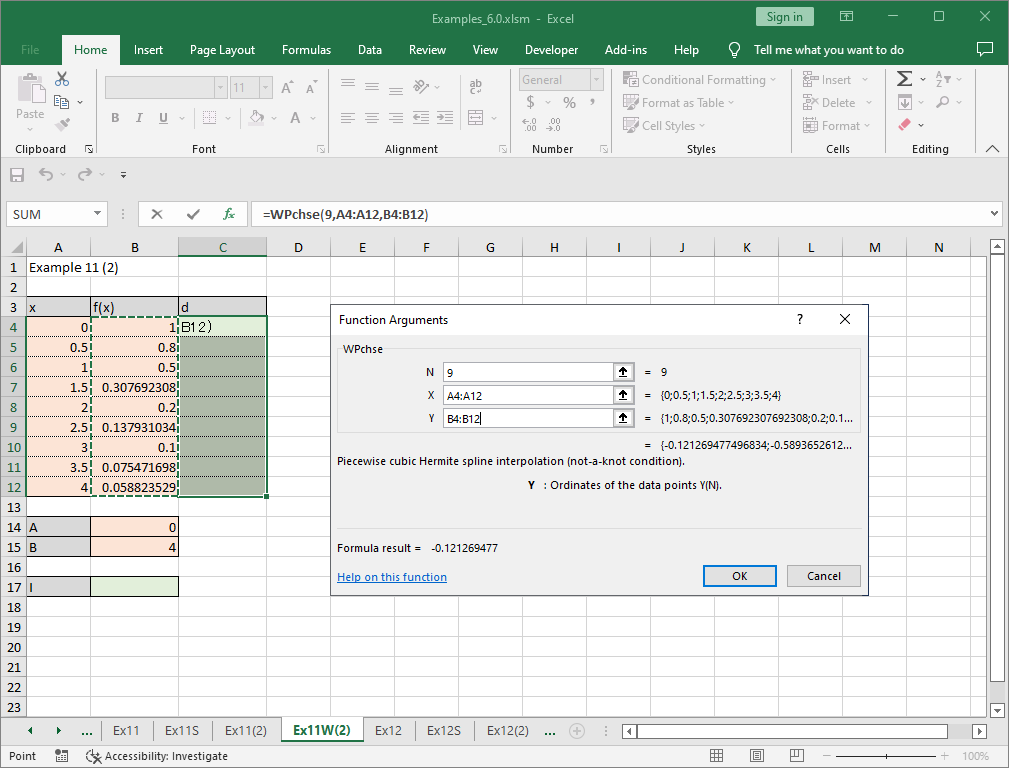Once coefficients are computed, integral value can be computed by worksheet function WPchia. The parameters required by WPchia are A, B, N, X, Y and D. A and B are the integral interval (= 0 and 4 for this example). N is the number of data (= 9 for this example). X and Y are the cell range of interpolated data. D is the cell range of spline coefficients.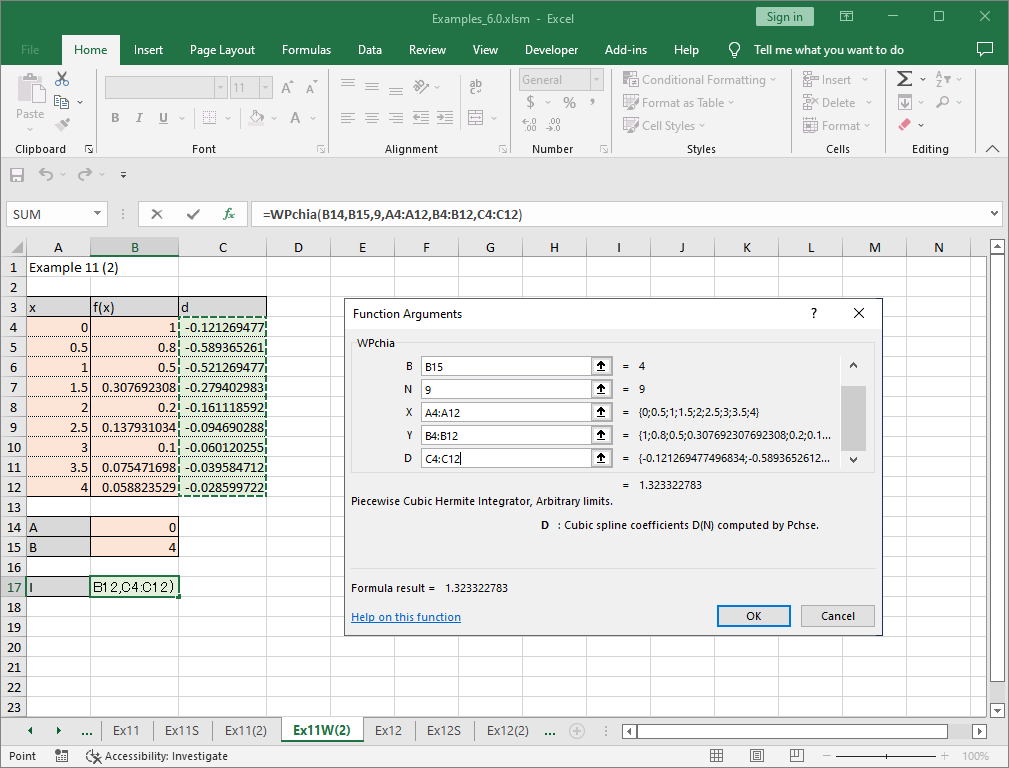Press Enter, then computed value 1.3233228 is obtained with 3 digit accuracy from 9 point data.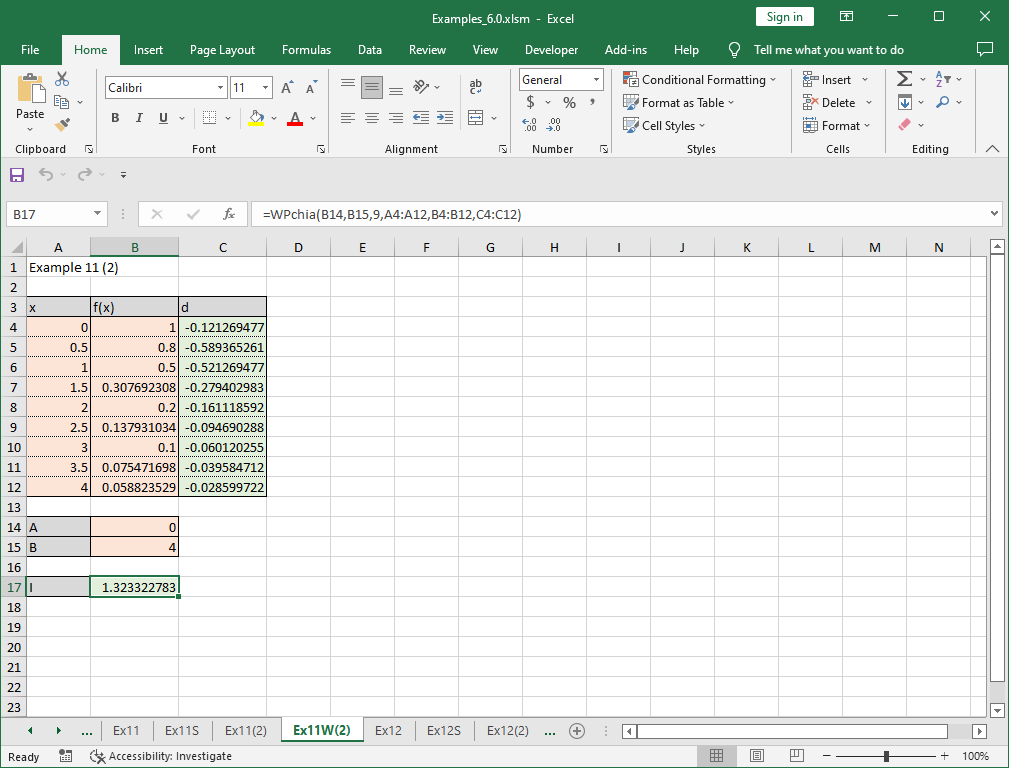### Solving problem with Solver

“Quadrature (finite interval)” of XLPack solver add-in can be used to solve this problem. The formulas (=1/(1+B3^2)) is entered in cell B4.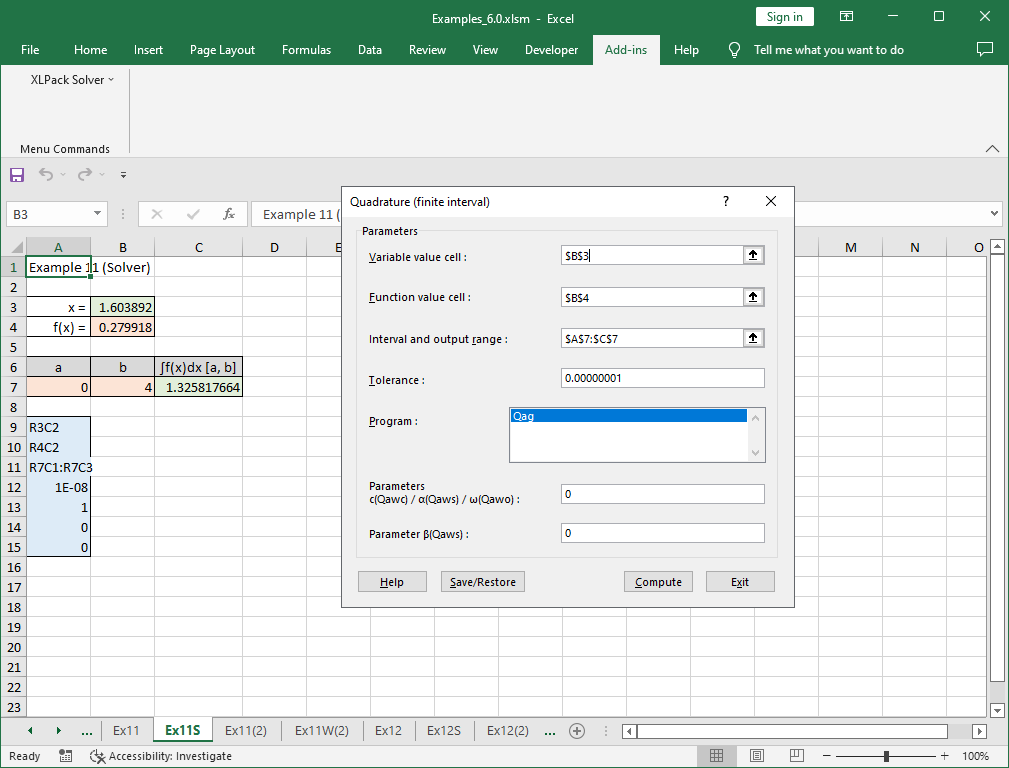Please refer to here for how to use solver.